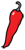The Grand Locus / Life for statistical sciences

## The reverend’s gambitTwo years after the death of Reverend Thomas Bayes in 1761, the famous theorem that bears his name was published. The legend has it he felt the devilish nature of his result and was too afraid of the reaction of the Church to publish it during his lifetime. Two hundred and fifty years later, the theorem still sparkles debate, but among statisticians.

Bayes theorem is the object of the academic fight between the so-called frequentist and Bayesian schools. Actually, more shocking than this profound disagreement is the overall tolerance for both points of view. After all, Bayes theorem is a theorem. Mathematicians do not argue over the Pythagorean Theorem: either there is a proof or there isn’t. There is no arguing about that.

So what’s wrong with Bayes theorem? Well, it’s the hypotheses. According to the frequentist, the theorem is right, it is just not applicable in the conditions used by the Bayesian. In short, the theorem says that if $A$ and $B$ are events, the probability of $A$ given that $B$ occurred is $P(A|B) = P(B|A) P(A)/P(B)$. The focus of the fight is the term $P(B)$. For the frequentist, $B$ has to be the outcome of a defined random experiment, like drawing a ball from an urn, polling a population at random etc. No random experiment, no probability, and in that case the theorem does not apply. For the Bayesian, $B$ always has a probability and therefore the theorem always applies.

This concept is very important so let’s clarify it. Christian Robert in The Bayesian Choice expresses the Bayesian credo in the following terms:

Proposing a distribution on the unknown parameters of a statistical model can be characterized as a probabilization of uncertainty, that is, as an axiomatic reduction from the notion of unknown to the notion of random.

In fine this is all about the definition of randomness. For the frequentist, randomness is the outcome of a repeatable experiment and for the Bayesian, randomness is a subjective measure of ignorance. As such, it is no problem to give $P(B)$ an arbitrary value, which is called the prior in Bayesian jargon.

So who’s right? Well, that’s the one million dollar question. As a student, I inclined towards the frequentist, as I disliked the arbitrariness of the prior. This is the weak point of Bayesian statistics, but overall, the Bayesian decision theory is much more consistent than the frequentist approach. For example, most statisticians will agree that the Gaussian distribution, say, is at best a useful approximation of the phenomenon under study. However, in the frequentist paradigm, the mean and the variance of this approximate model are considered perfectly accurate, non random numbers...

In my last post about p-values, I argued that the use of p-values is dangerous because it transmits a false idea of magnitude. The last comment about the frequentist approach raises an even stronger issue about p-values (which are the ultimate incarnation of the frequentist approach) namely that they naturally vanish when the sample size increases. Did I just write that for a large enough sample size, every test is significant? I guess I just did.

James Berger in Statistical Decision Theory and Bayesian Analysis gives the following example: say we want to test whether a Gaussian distribution has 0 mean, while it actually is 10-10. For a sample size of 1024, it is almost certain that the null hypothesis will be rejected. Because statistical models are approximations, the same goes for their parameters, so for every test there exists a sample size large enough so that rejection is almost certain.

This was not a big issue when data and testing were expensive. But today, with the advent of computers and high throughput technologies the trend becomes apparent. In a few years of R sessions at the computer I have never seen a p-value higher than 0.05 with real life datasets of sample size 10,000 or more, which is not so large for genomics.

Perhaps the time is right for a serious re-evaluation of the statistical paradigm in biological science.